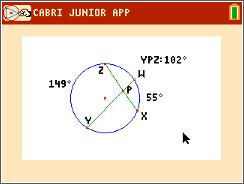### Mathematics lessons for IB® Diploma Programme

Applications and Interpretation | Geometry and Trigonometry

# Circles: Angles and Arcs

### Objectives• Students will define and identify central angles, major and minor arcs, intercepted arcs, and inscribed angles of a circle.
• Students will determine and apply the following relationships:
• Two inscribed angles intercepting the same arc have the same measure.
• An inscribed angle measure of 90° results in the endpoints of the intercepted arc lying on a diameter.
• The measure of an angle inscribed in a circle is half the measure of the central angle that intercepts the same arc.
• Students will try to make a connection with how to understand these topics in IB Mathematics courses on their final assessments

### Vocabulary

• Central angle
• Inscribed angle
• Major arc
• Minor arc
• Intercepted arc